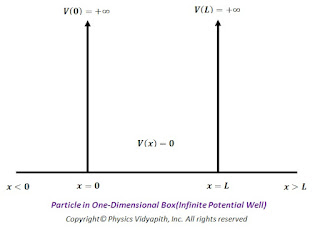### Particle in one dimensional box (Infinite Potential Well)

Let us consider a particle of mass $m$ that is confined to one-dimensional region $0 \leq x \leq L$ or the particle is restricted to move along the $x$-axis between $x=0$ and $x=L$. Let the particle can move freely in either direction, between $x=0$ and $x=L$. The endpoints of the region behave as ideally reflecting barriers so that the particle can not leave the region. A potential energy function $V(x)$ for this situation is shown in the figure below.Particle in One-Dimensional Box(Infinite Potential Well)
The potential energy inside the one -dimensional box can be represented as

$\begin{Bmatrix} V(x)=0 &for \: 0\leq x \leq L \\ V(x)=\infty & for \: 0> x > L \\ \end{Bmatrix}$

$\frac{\partial^{2} \psi(x)}{\partial x^{2}}+\frac{2m}{\hbar^{2}}(E-V)\psi(x)=0 \qquad(1)$

If the particle is free in a one-dimensional box, Schrodinger's wave equation can be written as:

$\frac{\partial^{2} \psi(x)}{\partial x^{2}}+\frac{2mE}{\hbar^{2}}\psi(x)=0$

$\frac{\partial^{2} \psi(x)}{\partial x^{2}}+\frac{8 \pi^{2} mE}{h^{2}}\psi(x)=0 \quad (\because \hbar=\frac{h}{2 \pi}) \quad(2)$

$\frac{\partial^{2} \psi(x)}{\partial x^{2}}+ k^{2}\psi(x)=0 \quad (\because k^{2}=\frac{8 \pi^{2} mE}{h^{2}}) \quad(3)$

The general solution of the above differential equation $(2)$

$\psi(x)= A sin(kx)+ B cos(kx) \qquad(4)$

The wave function $\psi(x)$ should be zero everywhere outside the box since the probability of finding the particle outside the box is zero. Similarly, the wave function $\psi(x)$ must also be zero at walls of the box because the probability density $[\psi(x)]^{2}$ must be continuous. Thus, the boundary conditions for this problem is that

(i) $\psi(x)=0$ For $x=0$

(ii) $\psi(x)=0$ For $x=L$

Now applying the boundary condition in equation$(4)$ i.e.

(i) At $x=0$ the wave function $\psi(0)=0$

Now we get

$\psi(0)= A sin(k.0)+ B cos(k.0)$

$A sin(k.0)+ B cos(k.0)=0 \qquad (\because \psi(0)= 0)$

$B=0$

Hence substitute the value of $B$ in equation$(4)$ ,

$\psi(L)= A sin(kx) \qquad(5)$

Now applying the second boundary condition:

(ii) At $x=L$ the wave function $\psi(L)=0$, we get

$\psi(x)= A sin(kL) \qquad(6)$

This equation will satisfy only for certain values of $k$, say $k_{n}$. Since $A$ can not be taken zero hence

$sin(k_{n}L)=0$

$sin(k_{n}L)=sin(n\pi)$

$k_{n}L=n\pi$

$k_{n}=\frac{n\pi}{L} \qquad(7)$

Thus for each allowed values of $k_{n}$ there is a wave function $\psi(x)$ given as, using equation$(5)$ and equation$(7)$

$\psi_{n}(x)=A sin(\frac{n\pi x}{L})$

This is the expression of the wave function or eigen function for a particle in a box.

Now, from equation $(3)$ and equation$(7)$, we get

$k^{2}=\frac{8 \pi^{2} mE}{h^{2}}= (\frac{n \pi}{L})^{2}$

$E=\frac{n^{2} h^{2}}{8mL^{2}}$

This is the expression of energy or eigen value for a particle in a box.

In general, the expression for this energy is written as:

$E_{n}=\frac{n^{2} h^{2}}{8mL^{2}}$

For different values of $n$ energy values can be written as

For $n=1$

$E_{1}=\frac{h^{2}}{8mL^{2}}$

It is known as zero-point energy or ground energy state

For $n=2$

$E_{2}=\frac{2^{2} h^{2}}{8mL^{2}}=2^{2}E_{1}$

For $n=3$

$E_{3}=\frac{3^{2} h^{2}}{8mL^{2}}=3^{2}E_{1}$

For $n=4$

$E_{4}=\frac{4^{2} h^{2}}{8mL^{2}}=4^{2}E_{1}$

So generalized form of the above equation can be written as

$E_{n}=n^{2}E_{1}$

Some of the possible energies for a particle in a box are shown on an energy-level diagram in the figure below.Possible Energies for a particle in a box
The energy levels have a spacing that increases with increasing $n$ and thus the particle in a box can take only certain discrete energy values, called Eigen-values. This means that the energy levels of a particle in a box are quantized but according to classical mechanics, the particle may take any continuous range of energy values between zero and infinity.